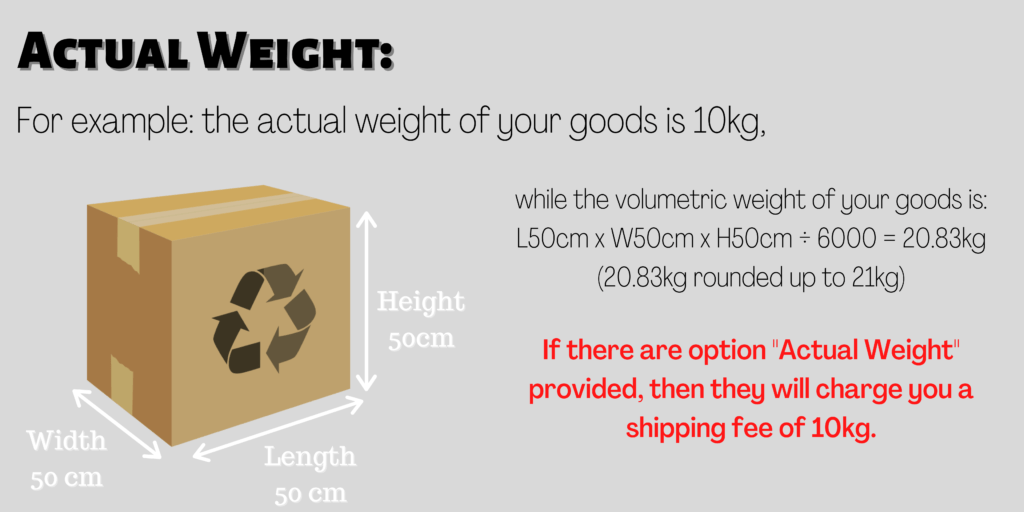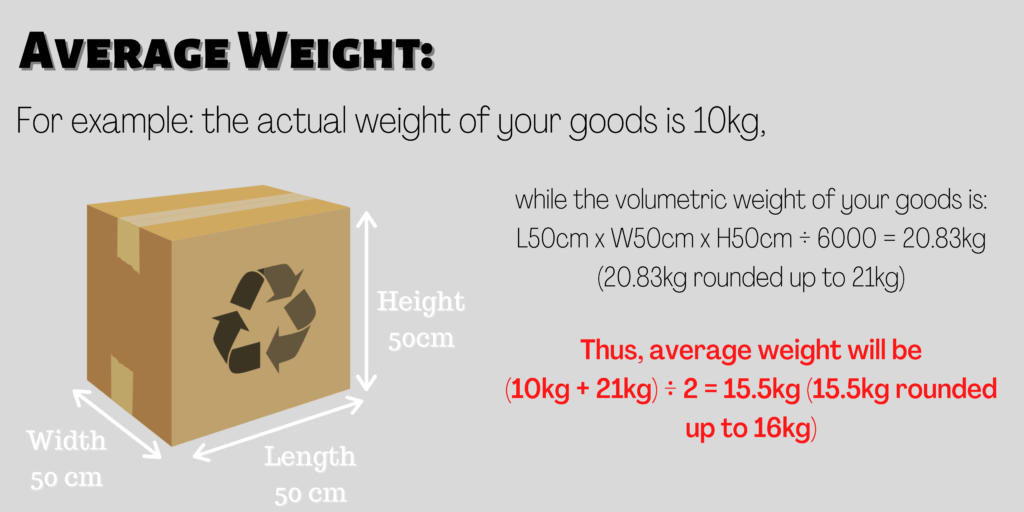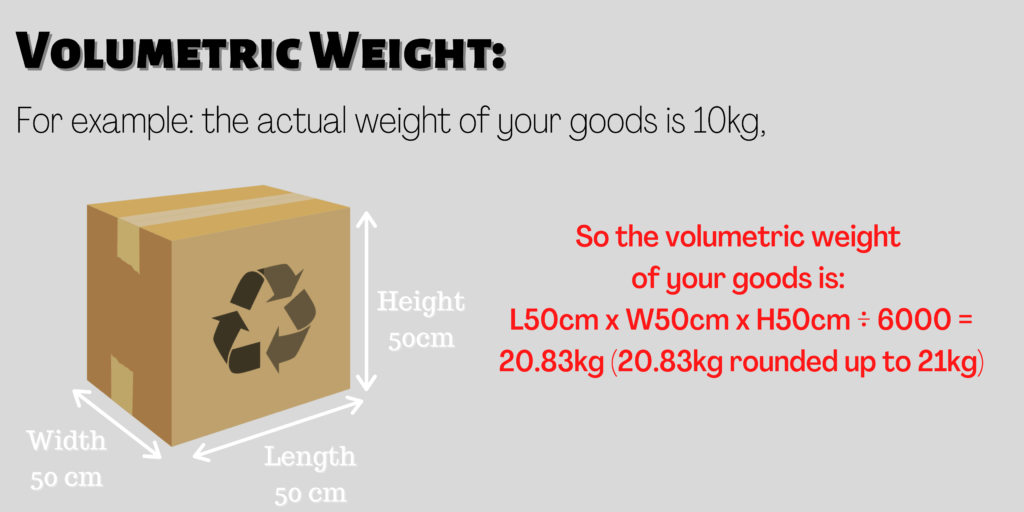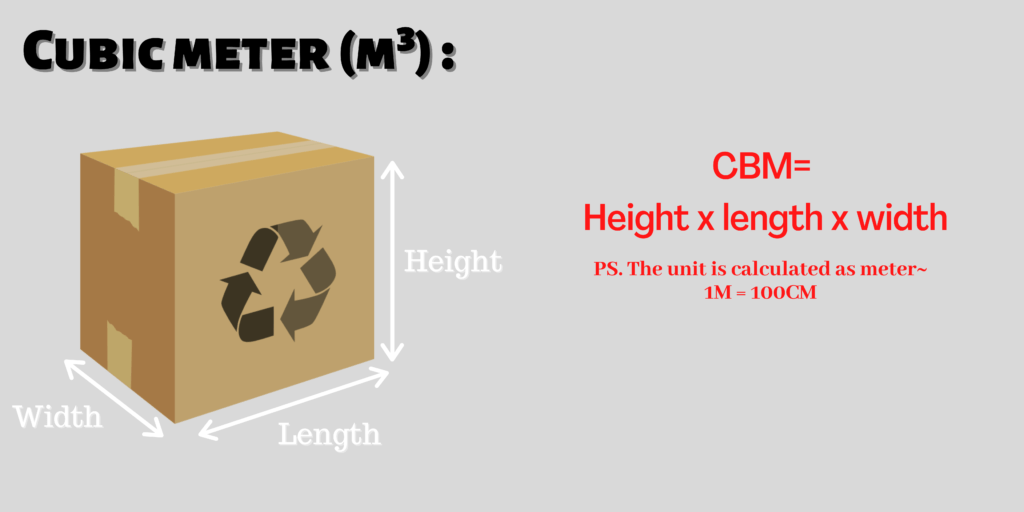# 4 Measurement methods of cargoWhenever you consult logistic customer service regarding the weight of your goods, you will encounter some specific freight calculation terms. There are four well-known calculation terms for cross-border logistic freight. For instance, the four terms are actual weight, average weight, volumetric weight, and cubic meter. By all means, today we will show you the differences between these measurement methods of cargo.

### Actual Weight:

The calculation takes the actual weight of the goods instead of the volumetric weight. Hence, the shipping fee will be calculated based on the actual weight of the goods.For example the actual weight of your goods is 10kg,

while the volumetric weight of your goods is: L50cm x W50cm x H50cm ÷ 6000 = 20.83kg (20.83kg rounded up to 21kg)

Provided that they do not provide the option of “actual weight”, they will charge you a shipping fee of 21kg.

In case they do provide the option of “actual weight”, they will charge you a shipping fee of 10kg.

### Average Weight：

The shipping fee will be calculated based on the average between the actual weight and the volumetric weight of the goods.For example: the actual weight of your goods is 10kg，

while the volumetric weight of your goods is: L50cm x W50cm x H50cm ÷ 6000 = 20.83kg (20.83kg rounded up to 21kg)

Thus the average weight will be:

(10kg + 21kg) ÷ 2 = 15.5kg (15.5kg rounded up to 16kg)

##### Formula

Average Weight= (Actual Weight + Volumetric Weight) ÷  2

Provided that they do not provide the option of “average weight”, they will charge you a shipping fee of 23kg.

In case they do provide the option of “average weight”, they will charge you a shipping fee of 16kg.

### Volumetric Weight：

The calculation takes the heaviest weight between the actual weight and the volumetric weight.For example: the actual weight of your goods is 10kg，

while the volumetric weight of your goods is: L50cm x W50cm x H50cm ÷ 6000 = 20.83kg (20.83kg rounded up to 21kg)

##### Formula

Volumetric Weight = L x W x H ÷ 6000

Provided that they do not provide the option of “volumetric weight”, they will charge you a shipping fee of 10kg.

In case they do provide the option of “volumetric weight”, they will charge you a shipping fee of 21kg.

### Cubic meter (m³):

It is calculated based on volume units of a cube with edges one meter in length. In general, one meter on each side is equal to one cubic meter.Nevertheless, 1CBM cubic meter can hold up to maximum weight of 500kg. It is often used as calculation method for sea freight large shipment.

So how much is one cubic meter?

To point out, 1CBM cubic meter is equal to 1M x 1M x 1M.

##### Formula

CBM = L(M) x W(M) x H(M)

#### Summary

 Actual Weight Volumetric Weight Average Weight Cubic Meter Description Calculate on actual weight Calculate the heaviest between actual weight and volumetric weight Calculate the average weight when volumetric weight is heavier than actual weight Calculate on cubic meter Calculation method Measure actual weight (L X W X H)/6000 = Volumetric Weight (Actual Weight+ Volumetric Weight) / 2 L(M) X W(M) X H(M) =m³ Commonly used at Air freight, Sea freight small parcel Air freight, Sea freight small parcel Air freight, Sea freight small parcel Sea freight large parcel

In overall, I hope that now you have a better understanding of the freight measurement methods of logistics cargo. However, do note that different logistics companies will use the above calculation methods based on the situation. With this in mind, if you want to know more about the calculation methods of cargo, please feel free to contact our customer service.Click here for more import terminologies3 must-known import terminologiesFor more details, visit us at our Facebook page or register with us and get in contact

Parcel consolidation 👉🏻

Sea freight large parcel 👉🏻